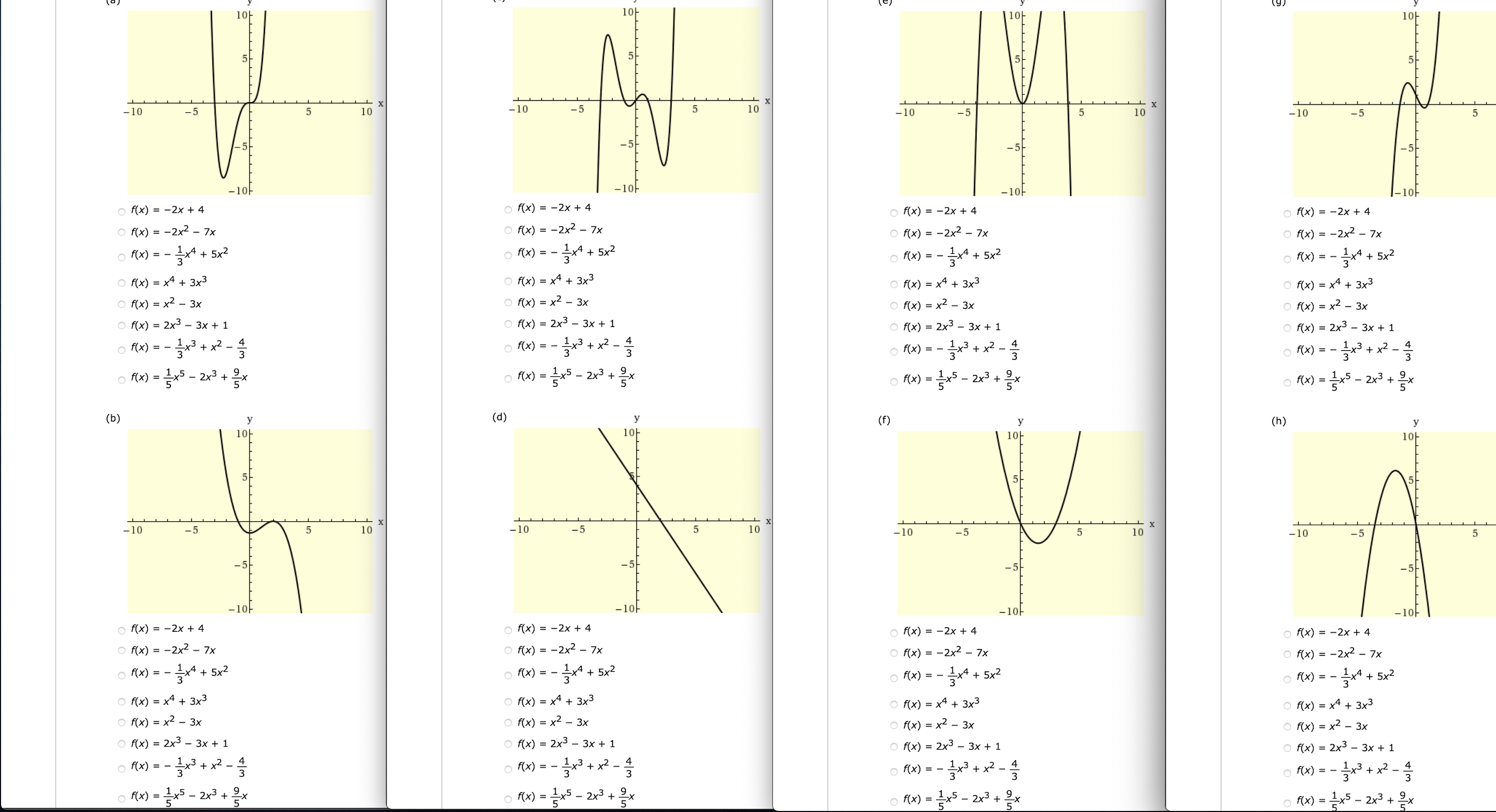# (9)10H10-10F-10-510-10.510-1010-5-10-5-10F-10--10-10FO f(x) = -2x + 4o f(x) = -2x + 4o f(x) = -2x + 4O f(x) = -2x + 4O f(x) = -2x2 – 7x-2x2 - 7xO f(x)O f(x) = -2x2-2x2- 7xO f(x) =7x5x2-* + 5x2o f(x) = - *o f(x) = -+ 5x23O f(x)+ 5x2o f(x)3O f(x) = x4 + 3x3O f(x) = x4 + 3x³O f(x) = x4 + 3x3O f(x) = x4 + 3x3O f(x) = x2 –O f(x) = x2 - 3x- 3xO f(x) = x2- 3xO f(x) = x2- 3xO f(x) = 2x3 - 3x + 12x3 – 3x + 1O f(x)2x3O f(x)O f(x) = 2x3 - 3x + 1- 3x + 1%3Do Ax) = -o Pa) =+ x? -4+ x? -+ x2 -3o Rx) = -+ x23O f(x)+ x2 -O f(x)= -3- 2r3 + xo fx) =- 2x3 +x9.-хo (x) = 5 - 2x3 +O f(x) =(d)(b)y(f)У(h)yy10-10-10-10х-10-51010-10-5-10-10-5-5-10--10F-10--10-o f(x) = -2x + 4o f(x) = -2x + 4O f(x) = -2x + 4o f(x) = -2x + 4O f(x) = -2x2 – 7xO f(x) = -2x² – 7xO f(x)-2x27xO f(x) = -2x2- 7x1,4 + 5x23글 + 5x2o f(x)o f(x) = -O f(x)+ 5x25x2O f(x) = -O f(x) = x4 + 3x3O f(x) = x4 + 3x3O f(x) = x4 + 3x3O f(x) = x4 + 3x3O f(x) = x2 - 3xO f(x) = x2 - 3xO f(x) = x2- 3x· 3xO f(x) = x22x3 - 3x + 1O f(x) = 2x3 - 3x + 1O f(x)O f(x) = 2x3 - 3x + 1O f(x) = 2x3 - 3x + 1%3Do Rw) = - * -o M) = -Rx) = -+ x2 -4+ x2O (x)4+ x² -O f(x)O f(x)333x5 - 2x3 +.2x39.o f(x)RA) =5 - 2x3 +.O Fx) = 5 - 2x3O (x) =O f(x)-5

Question
14 views

Match the polynomial function with its graph: [A] through [H]help_outlineImage Transcriptionclose(9) 10H 10- 10F -10 -5 10 -10 .5 10 -10 10 -5 -10 -5 -10F -10- -10 -10F O f(x) = -2x + 4 o f(x) = -2x + 4 o f(x) = -2x + 4 O f(x) = -2x + 4 O f(x) = -2x2 – 7x -2x2 - 7x O f(x) O f(x) = -2x2 -2x2 - 7x O f(x) = 7x 5x2 -* + 5x2 o f(x) = - * o f(x) = - + 5x2 3 O f(x) + 5x2 o f(x) 3 O f(x) = x4 + 3x3 O f(x) = x4 + 3x³ O f(x) = x4 + 3x3 O f(x) = x4 + 3x3 O f(x) = x2 – O f(x) = x2 - 3x - 3x O f(x) = x2 - 3x O f(x) = x2 - 3x O f(x) = 2x3 - 3x + 1 2x3 – 3x + 1 O f(x) 2x3 O f(x) O f(x) = 2x3 - 3x + 1 - 3x + 1 %3D o Ax) = - o Pa) = + x? - 4 + x? - + x2 - 3 o Rx) = - + x2 3 O f(x) + x2 - O f(x) = - 3 - 2r3 + x o fx) = - 2x3 +x 9. -х o (x) = 5 - 2x3 + O f(x) = (d) (b) y (f) У (h) y y 10- 10- 10- 10 х -10 -5 10 10 -10 -5 -10 -10 -5 -5 -10- -10F -10- -10- o f(x) = -2x + 4 o f(x) = -2x + 4 O f(x) = -2x + 4 o f(x) = -2x + 4 O f(x) = -2x2 – 7x O f(x) = -2x² – 7x O f(x) -2x2 7x O f(x) = -2x2 - 7x 1,4 + 5x2 3 글 + 5x2 o f(x) o f(x) = - O f(x) + 5x2 5x2 O f(x) = - O f(x) = x4 + 3x3 O f(x) = x4 + 3x3 O f(x) = x4 + 3x3 O f(x) = x4 + 3x3 O f(x) = x2 - 3x O f(x) = x2 - 3x O f(x) = x2 - 3x · 3x O f(x) = x2 2x3 - 3x + 1 O f(x) = 2x3 - 3x + 1 O f(x) O f(x) = 2x3 - 3x + 1 O f(x) = 2x3 - 3x + 1 %3D o Rw) = - * - o M) = - Rx) = - + x2 - 4 + x2 O (x) 4 + x² - O f(x) O f(x) 3 3 3 x5 - 2x3 +. 2x3 9. o f(x) RA) = 5 - 2x3 +. O Fx) = 5 - 2x3 O (x) = O f(x) - 5 fullscreen
check_circle

star
star
star
star
star
1 Rating
Step 1

Since we only answer up to 3 sub-parts, we’ll answer the first 3. Please resubmit the question and specify the other subparts (up to 3) you’d like answered

Step 2

Given, graphs

Part (a):

Step 3

The x – intercept of graph at x = -3 and x = 0. From graph, the minimum value of function occu...

### Want to see the full answer?

See Solution

#### Want to see this answer and more?

Solutions are written by subject experts who are available 24/7. Questions are typically answered within 1 hour.*

See Solution
*Response times may vary by subject and question.
Tagged in

### Calculus